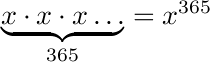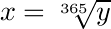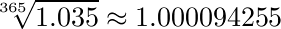## Even more interesting

Posted by – February 17, 2008

I was reading through the terms and conditions of a savings account I have, and in the part where the calculation of interest is explained it says “The interest on capital is computed daily, with the number 360 used as the denominator.” Huh? Denominator? And aren’t there 365 days a year? Does this mean I’m missing out on five days of interest a year? I needed to know! But perhaps not badly enough, because I forgot about it until yesterday evening when I was trying to get to sleep and the answer suddenly occurred to me.

Now, the interest of the account is given as an effective annual interest, meaning that it is computed in some way that gives the same result as a certain interest compunded once a year, assuming that the capital doesn’t change. Of course, the capital does sometimes change, so they’ve chosen to calculate a daily interest that matches the promised annual interest instead. If the interest is such that it multiplies the capital by x, after one year the capital has effectively become multiplied byFor that to be equivalent to some annual interest multiplier y, necessarilySo the desired daily interest is the three-hundred-and-sixty-fifth root of the annual interest. Now, that’s not something you’d necessarily want to work out with a piece of paper, but a calculator can do it in an instant. For instance, the current annual interest of the account in question is 3.5% so to compound daily the multiplier would bewhich corresponds to an interest of about 0.0094%. Okay, so that’s the correct way of finding an appropriate daily interest (in the sense that it’s equivalent to the definition). What could the bank possibly be doing with denominators and the number 360? Could 3.5% divided by 360 be approximately the 365th root of 1.035 minus one? Let’s see:It is! So I’m guessing this is what the bank actually does. Notice that their method even produces a slightly higher result. Does this result in any actual difference over a year?Yes! I’m up a tenth of a percentage point on this deal! Big money, here I come.

I can understand that in olden days it might have been a bit onerous to calculate 365th roots of ever-changing interests, so someone thought of this alternative method. For small interests it’s only off by a bit. But today, in the time of practically free computing power? Seems a bit odd.

edit: I just realised something: not all years have 365 days! Allowing for leap years, the average year has 365 + 97/400 = 365.2425 days, so I should really have been taking the 365.2425th root and also using that figure for finding the difference between the bank’s method and the correct method, but it doesn’t really change anything.

edit the second: now that I read over the details again, it seems to imply that the interest is calculated daily but not compounded to the capital. So they’re doing something else I don’t want to get into right now. Banking is complicated.